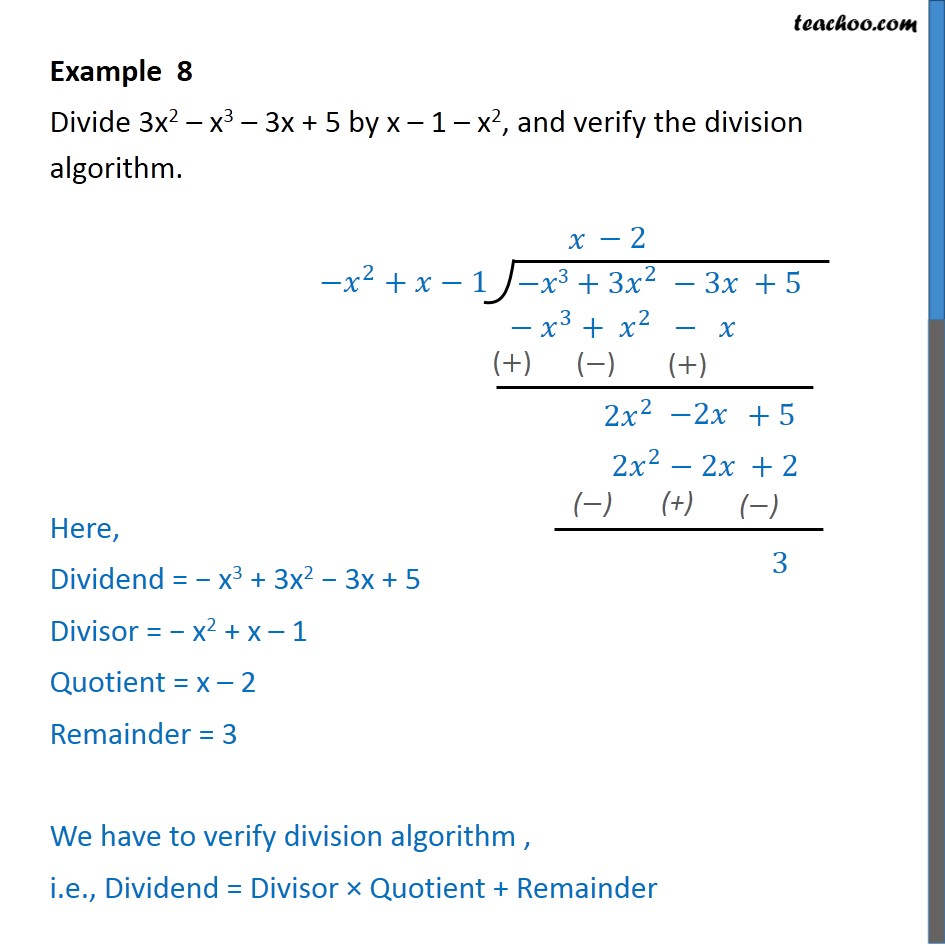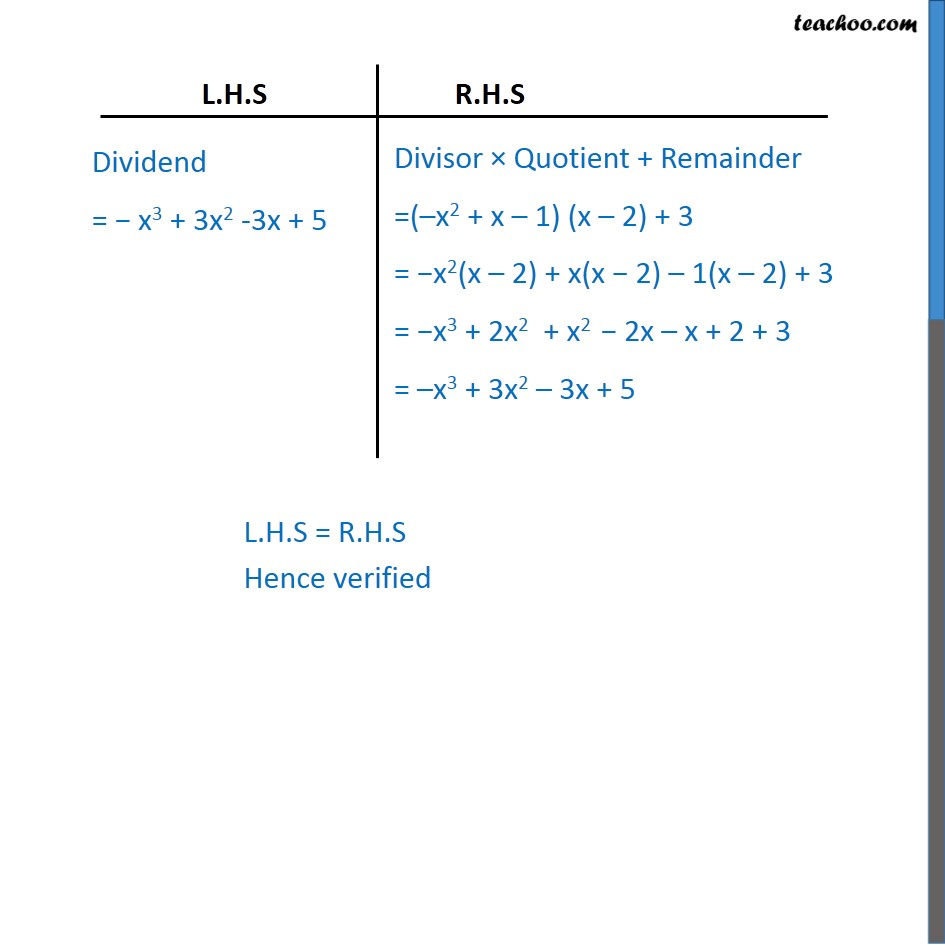Chapter 2 Class 10 Polynomials

Class 10
Important Questions for Exam - Class 10Learn in your speed, with individual attention - Teachoo Maths 1-on-1 Class

### Transcript

Example 8 Divide 3x2 – x3 – 3x + 5 by x – 1 – x2, and verify the division algorithm. Here, Dividend = − x3 + 3x2 − 3x + 5 Divisor = − x2 + x – 1 Quotient = x – 2 Remainder = 3 We have to verify division algorithm , i.e., Dividend = Divisor × Quotient + Remainder